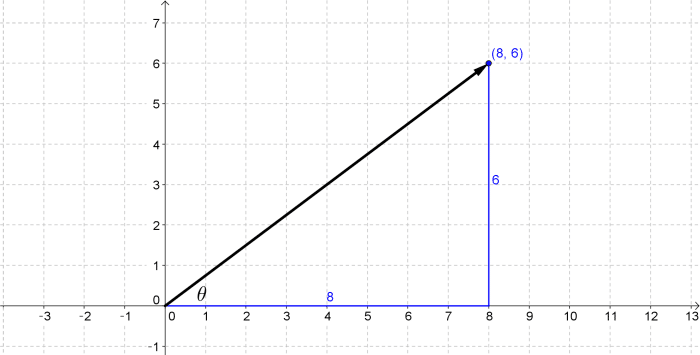# What Is The Angle Between The Given Vector And The Positive Direction Of The X-axis

We thoroughly check each answer to a question to provide you with the most correct answers. Found a mistake? Let us know about it through the REPORT button at the bottom of the page.

What is the angle between the given vector and the positive direction of the x-axis? 8i + 6j

## Explanation8i + 6j

Draw a vector from the origin to the point (8,6).

The angle is between the positive x-axis and the vector.

tan0 = 6/8 = 3/4

0 = arctan3/4 = 36.87.